# KSEEB Solutions for Class 10 Maths Chapter 3 Pair of Linear Equations in Two Variables Ex 3.7

Students can Download Class 10 Maths Chapter 3 Pair of Linear Equations in Two Variables Ex 3.7 Questions and Answers, Notes Pdf, KSEEB Solutions for Class 10 Maths helps you to revise the complete Karnataka State Board Syllabus and score more marks in your in examinations

## Karnataka State Syllabus Class 10 Maths Chapter 3 Pair of Linear Equations in Two Variables Ex 3.7

Question 1.
The ages of two friends Ani and Biju differ by 3 years. Ani’s father Dharam is twice as old as Ani and Biju is tw ice as old as his sister Cathy. The ages of Cathy and Dharam differ by 30 years. Find the ages of Ani and Biju.
Let the ages of Ani and Biju be ‘x’ years and ‘y’ years respectively.
x – y = 3 (x > y) (or) y – x = 3 (y > x) → (1)
Age of Ani’s father Dharm = 2x years
Age of Biju’s sister = $$\frac{y}{2}$$ years.
According to the Question
2x – $$\frac{y}{2}$$ = 30
4x – y = 60 → (2)
case (i) when x – y = 3
x – y= 3 → (1)
4x – y = 60 → (2)
Subtract equation (1) and (2)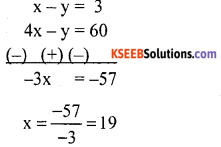x = 19 years
Put x = 19 in equation (1)
x – y = 3
19 – y = 3
– y = 3 – 19
y = 16
Ani’s age = x = 19 years.
Biju’s age = y = 16 years.
case (ii) y – x = 3
– x + y = 3 → (1)
4x – y = 60 → (2)x = 21
Put x = 21 in equation (1)
– 21 + y = 3
y = 3 + 21
y = 24
Ani’s age is 21 years and Biju age is 24 years.Question 2.
One says, “Give me a hundred, friend! I shall then become twice as rich as you”. The other replies, “If you give me ten, I shall be six times as rich as you”. Tell me what is the amount of their (respective) capital? [From the Bijaganita of Bhaskara II
[Hint: x + 100 = 2(y – 100), y + 10 = 6(x – 10)].
Let the amount of their respective capitals be ₹x and ₹y
x + 100 = 2(y – 100)
x+ 100 = 2y – 200
x – 2y = – 300 → (1)
and y + 10 = 6 (x – 10)
y + 10 = 6x – 60
– 6x + y = – 70 multiply by – 2
12x – 2y = 140 → (2)
Subtract equation (1) and (2)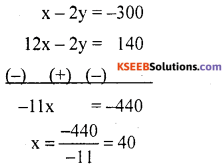x = 40
Put x = 40 in equation (1)
40 – 2y = – 300
– 2y = – 300 – 40
– 2y = – 340
y = $$\frac{340}{2}$$
y = 170
∴ amounts of their respective capitals are ₹40 and ₹170.

Question 3.
A train covered a certain distance at a uniform speed. If the train would have been 10 km/h faster, it would have taken 2 hours less than the scheduled time. And, if the train were slower by 10 km/h; it would have taken 3 hours more than the scheduled time. Find the distance covered by the train.
Let the actual speed of the train be x km/hr and the actual time taken be ‘y’ hours.
Distance = speed x time = x × y
= xy km
xy = (x + 10) (y – 2)
xy = xy – 2x + 10y – 20
2x- 10y = – 20
xy = (x – 10) (y + 3)
xy = xy + 3x – 10y – 30
3x – 10y = 30 → (2)
Subtract equation (1) and (2)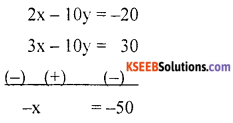Put x = 50 in equation (1)
2(50) – 10y = – 20
– 10y = – 20 – 100
– 10y = – 120
y = $$\frac{-120}{-10}$$
y = 12
distance covered by train = xy
= 50 × 12 = 600 km.Question 4.
The students of a class are made to stand in rows. If 3 students are extra in a row, there would be 1 row less. If 3 students are less in a row, there would be 2 rows more. Find the number of students in the class.
Let the number of students in the class be x and the number of rows be y. Then the number of students in each row $$\frac{x}{y}$$ .
If 3 students are extra in row, then there would be 1 row less i,e when each row
has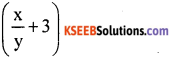Students then the number of rows is (y – 1)
∴ Total number of students
= number of rows x number of students in each row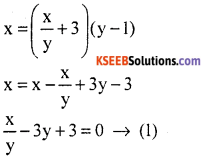and, if 3 students are less in a row, then there would be 2 rows more i.e, when each row has $$\left(\frac{x}{y}-3\right)$$ students, then the number of rows is (y + 2).
∴ Total number of students = Number of rows x number of students in each row.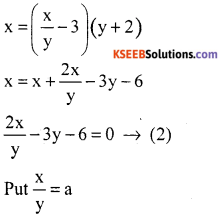Then equation (1) and (2) can be written as
a – 3y = – 3 → (3)
2a – 3y = 6 → (4)
Subtract equation (3) and (4)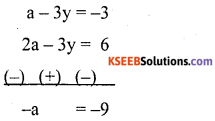a = 9
Put a = 9 in equation (3)
9 – 3y = – 3
– 3y = – 3 – 9
– 3 y = – 12
x = 9 × 4
x = 36
Hence number of students in class is 36 and number of rows is 4.

Question 5.
In a ∆ ABC, ∠C = 3 ∠B = 2 (∠A + ∠B). Find the three angles.
Given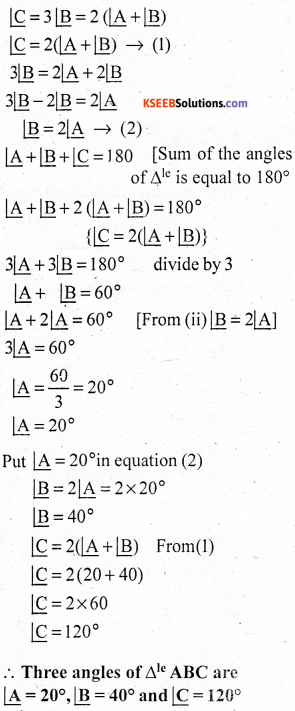Question 6.
Draw the graphs of the equations 5x – y = 5 and 3x – y = 3. Determine the coordinates of the vertices of the triangle formed by these lines and the y-axis.
5x – y = 5 → (1)
3x – y = 3 → (2)
From equation (1)
y = 5x – 5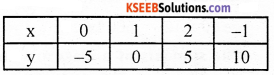From equation (2)
y = 3x – 3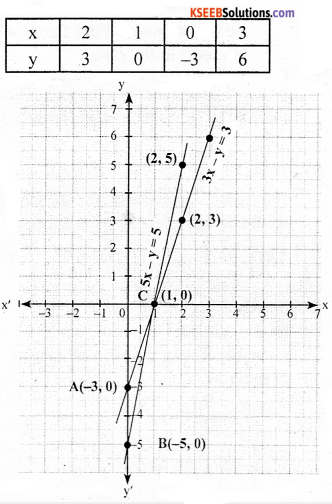In the graph, we observe that the coordinates of the vertices’s of the triangle ABC formed by the two lines represented, by the A(0, -3) and B(0, -5).Question 7.
Solve the following pair of linear equations:
(i) px + qy = p – q
qx – py = p + q
px + qy = p – q → (1)
qx-py = p + q → (2)
Multiply equation (1) p and equation (2) by q
p2x + pqy = p2 – pq → (3)
q2x – pqy = pq + q2 → (4)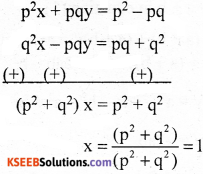Put x = 1 in equation (1).
p(1) + qy = p – q
qy = p – q – p
qy = – q
y = $$\frac{-q}{q}$$
y = – 1
Hence, the solution of the given pair of linear equation is x = 1, y = – 1

(ii) ax + by – c
bx + ay = 1 + c
ax + by = c
bx + ay = 1 + c
then ax + by – c = 0 → (1)
bx + ay – (1 + c) = 0 → (2)
Solve by cross multiplication method.Hence, the solution of the given pair of linear equation is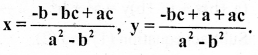ax + by = a2 + b2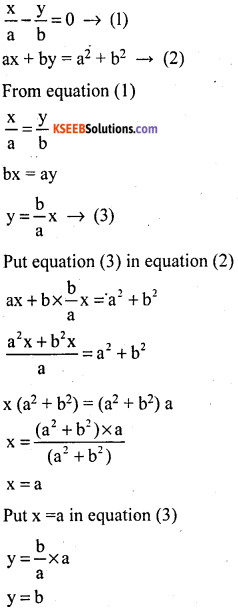Hence, the solution of the given pair of equations x = a, y = b.

(iv) (a – b)x + (a + b)y = a2 – 2ab – b2
(a + b) (x + y) = a2 + b2
(a – b)x + (a + b)y = a2 – 2ab – b2 → (1)
(a + b) (x + y) = a2 + b2
(a + b)x + (a + b)y = a2 + b2 → (2)
Subtract equation (1) and (2),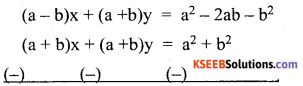(a – b)x – (a + b)x = a2 – 2ab – b2 – a2 – b2 ax – bx – ax – bx = – 2ab – 2b2.Put x = a + b in equation (1)
(a – b) (a + b) + (a + b)y = a2 – 2ab – b2
a2 – b2 + (a + b)y = a2 – 2ab – b2
(a + b)y = a2 – 2ab – b2 – a2 + b2
(a + b)y = – 2ab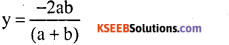Hence, solution of the pair of linear equation is x = a + b,(v) 152x – 378y = – 74
– 378x + 152y = – 604
152x – 378y = – 74 → (1)
– 378x + 152y = – 604 → (2)226x – 226y = – 678 divide by – 226
x + y = 3 → (3)
Subtract equation (1) and (2)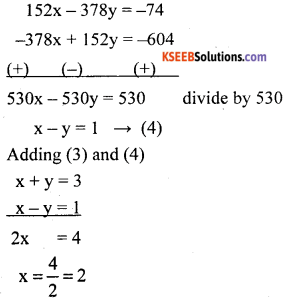Put x = 2 in equation (3)
2 + y = 3
y = 3 – 2
y = 1
Hence, the solution of the given pair of linear equations is x = 2, y = 1Question 8.
ABCD is a cyclic quadrilateral (see Fig.3.7). Find the angles of the cyclic quadrilateral.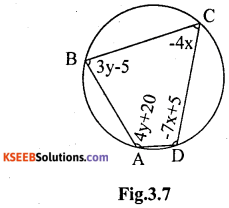We know that the opposite angles of a cyclic Quadrilateral are supplementary,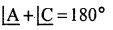4y + 20 (4x) = 180°
4y – 4x = 180 – 20
4y – 4x = 160 divide by 4
y – x = 40 → (1)3y – 5 + (- 7x + 5 ) = 180°
3y – 5 – 7x + 5 = 180°
3y – 7x = 180° → (2)
From equation (1)
y = 40 + x → (3)
Put equation (3) in equation (2)
3(40 + x) – 7x = 180°
120 + 3x – 7x = 180°
– 4x = 60°
x = $$\frac{60}{-4}$$
x = – 15
Put x = -15 in equation (1)
y – x = 40
y = 40 4 – x
y = 40 – 15
y = 25°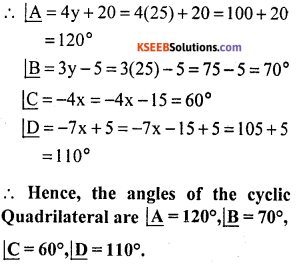error: Content is protected !!